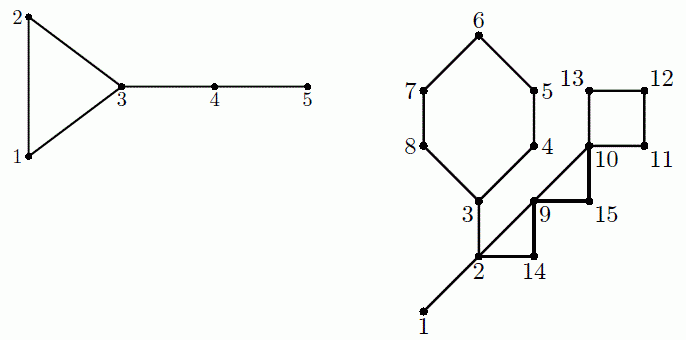Competitions

# Cactus Revenge

NE(E)RC featured a number of problems in previous years about cactuses — connected undirected graphs in which every edge belongs to at most one simple cycle. Intuitively, a cactus is a generalization of a tree where some cycles are allowed. The traditional cactus that was initially used in NEERC 2005 problem is given on the second picture in the Examples section.

You are given n integers d1, d2, ..., dn. Construct any cactus with n vertices such that vertex i has degree di (i. e. exactly di incident edges), or determine that no such cactus exists. Parallel edges and loops are not allowed.

#### Input

The first line contains a single integer n (2n2000) - the number of vertices in the cactus. The second line contains n integers d1, d2, ..., dn (1din - 1) - the desired vertex degrees.

#### Output

If it’s impossible to construct a cactus satisfying the conditions, output a single integer -1.

Otherwise, by tradition, output the constructed cactus as a set of edge-distinct paths.

In the first line print an integer m - the number of such paths. Each of the following m lines should contain a path in the graph. A path should start with an integer ki (ki2) followed by ki integers from 1 to n. These ki integers should represent consecutive vertices of this path. Adjacent vertices in the path should be distinct. The path can visit the same vertex multiple times, but every edge of the cactus should be traversed exactly once in the whole output.Time limit 1 second
Memory limit 128 MiB
Input example #1
5
2 2 3 2 1

Output example #1
5
2 2 4
2 2 3
2 1 3
2 3 5
2 1 4

Input example #2
4
3 3 2 2

Output example #2
-1

Input example #3
6
1 2 1 1 2 1

Output example #3
-1

Input example #4
15
1 4 3 2 2 2 2 2 4 4 2 2 2 2 2

Output example #4
18
2 9 11
2 9 10
2 2 10
2 2 3
2 3 4
2 2 4
2 5 9
2 5 6
2 6 9
2 7 10
2 7 8
2 8 10
2 1 3
2 11 12
2 12 13
2 13 14
2 14 15
2 2 15

Source 2019 ACM NEERC, Semifinals, December 1, Problem C